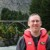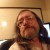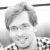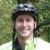How Can I Simulate Trial Execution Model for Virtual DoE?

Six Sigma – iSixSigma Forums General Forums Methodology How Can I Simulate Trial Execution Model for Virtual DoE?

Viewing 8 posts - 1 through 8 (of 8 total)
• Author
Posts
• #55858Gustav
Participant

I want to create a computer emulator for a learning purpose. It will emulate a binary outcome and use several Yes/No factors as an input (lets say A B C).
The output will be the % of successes.

The output when all factors are at “-” is 5% of successes.
When
A at “+” 5.5% (0.5% gain)
B at “+” 6.5% (1.5% gain)
C at “+” 5% (0% gain)
A and B at “+” (AB interaction) 7.5% (2.5% gain)

So I want to implement it in a software as a trial execution model with the list of random trials and outputs(depending on levels of factors A-B-C).

Example of software behavior:

Order of trial ABC Output
1st trial + + + Fail
2nd trial + – + Fail
3rd trial + – – Success
…..
10000 trial + – – Fail

I want it to look realistic so that not all the time the combination of A+ B- C- will result to “Success” because the process is not physical, it is a human response.

What is the general steps of constructing such an algorithm?
What is the most appropriate tool for this? Excel, JMP, R or simulation software like Anylogic, Promodel?

0
#201943Chris Seider
Participant

Excel or Minitab or any other ….

Unsure of where you’re going but it sounds interesting.

0
#201946Strayer
Participant

Why do you want to do this yourself? Configurable simulation software is readily available (for a price, of course). Even if you’re a talented computer scientist / software engineer this is a very difficult and time-consuming endeavor, you’re unlikely to do as well as what you can get “off the shelf”, and since you’re only looking at a specific scenario it won’t be useful for anything else.

0
#201947Sergey
Participant

It seems any software can help generate three sets of data according to your assumptions. Then you need to process it to get exact model. That’s why it is easier to do in software where you are running DOE analysis.

0
#201956Nik
Participant

If you know the formula, and how each X behaves (some typical value and variance), and you want to see how the results will behave… try a Monte Carlo Simulation. There is software for this, but you can use excel. Create column A, B, C, and a column Y for your formula (=A+B+A*B… whatever). Then for A, create a random number equation that has the same typical value and the distribution and create several thousand rows. Repeat for B, C, etc and fill your Y equation down.

This is probably easiest using MINITAB where they have numerous distributions and you quickly generate thousands of rows.

Google: “Understanding Monte Carlo Simulation with an Example” and click the Minitab Blog link.

0
#201967Liam
Participant

Yes, sounds like Monte Carlo would be a good fit here. I’d be happy to link you to an online SIMUL8 Simulation example that can do this. Can you explain more about the success criteria and the way that you want the randomization to occur? I.e. why is +-+ a fail and +– is a success? Should the variation in occurrence of these be totally random in each trial or set by % likelihood?

General steps would be
1) set your arrival behaviour; how many occurrences to test per trial, the likelihood of each variance + and -.
2) define you success criteria; usually we will do this with if statements or by using the ‘Route out by type’ function in SIMUL8.
3) have a means of recording results; in this case the success count or %.
4) Assess your trial results; in Monte Carlo typically by % occurrence.

0
#202415Gustav
Participant

Hi!

Sorry, I thought there were no new replies in the topic because I haven’t received any notifications.
Here is the JMP script that generates trials for full factorial:

New Table( "Untitled",
New Column( "A", Numeric, "Nominal", Format( "Best", 12 ),
Formula( Random Integer( 0, 1 ) ) ),
New Column( "B", Numeric, "Nominal", Format( "Best", 12 ),
Formula( Random Integer( 0, 1 ) ) ),
New Column( "C", Numeric, "Nominal", Format( "Best", 12 ),
Formula( Random Integer( 0, 1 ) ) ),
New Column( "Success?", Numeric, "Nominal", Format( "Best", 12 ),
Formula(
If(
:A == 0 & :B == 0 & :C == 0, Random Binomial( 1, 0.05 ),
:A == 0 & :B == 0 & :C == 1, Random Binomial( 1, 0.05 ),
:A == 0 & :B == 1 & :C == 0, Random Binomial( 1, 0.065 ),
:A == 0 & :B == 1 & :C == 1, Random Binomial( 1, 0.065 ),
:A == 1 & :B == 0 & :C == 0, Random Binomial( 1, 0.055 ),
:A == 1 & :B == 0 & :C == 1, Random Binomial( 1, 0.055 ),
:A == 1 & :B == 1 & :C == 0, Random Binomial( 1, 0.075 ),
:A == 1 & :B == 1 & :C == 1, Random Binomial( 1, 0.075 ),
Random Binomial( 1, 0.05 ) ) ) ) )

@nai102
, thank you. I will try to do the same with Monte Carlo (haven’t used it before).

@LiamH
, the model should be behavioral like reaction of a human on some combinations (product, ad, food), that’s why there should be variation. Not sure how much.
Is it possible to simulate responses in SIMUL8 for fractional factorial design? Now I am trying to do this.

0
#202416Gustav
Participant

Hi Sergei @ssobolev,
As far as I know only JMP has this functionality. I could simulate data in JMP for normal distribution but for binomial data it was difficult for me to translate the estimated effect sizes to coefficients that are need to be entered in Response Simulation dialog box.

0
Viewing 8 posts - 1 through 8 (of 8 total)

You must be logged in to reply to this topic.